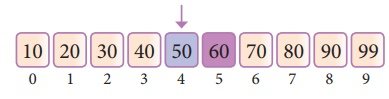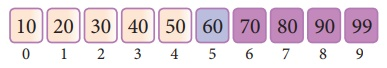Home | | Computer Science 12th Std | Algorithm for Searching Techniques

# Algorithm for Searching Techniques

Linear search also called sequential search is a sequential method for finding a particular value in a list.

Algorithm for Searching Techniques

## Linear Search

Linear search also called sequential search is a sequential method for finding a particular value in a list. This method checks the search element with each element in sequence until the desired element is found or the list is exhausted. In this searching algorithm, list need not be ordered.

### Pseudo code

1. Traverse the array using for loop

2. In every iteration, compare the target search key value with the current value of the list.

If the values match, display the current index and value of the array

If the values do not match, move on to the next array element.

3. If no match is found, display the search element not found.

To search the number 25 in the array given below, linear search will go step by step in a sequential order starting from the first element in the given array if the search element is found that index is returned otherwise the search is continued till the last index of the array. In this example number 25 is found at index number 3.Example 1:

Input: values[] = {5, 34, 65, 12, 77, 35}

target = 77

Output: 4

Example 2:

Input: values[] = {101, 392, 1, 54, 32, 22, 90, 93}

target = 200

## Binary Search

Binary search also called half-interval search algorithm. It finds the position of a search element within a sorted array. The binary search algorithm can be done as divide-and-conquer search algorithm and executes in logarithmic time.

### Pseudo code for Binary search

If the search element is equal to the middle element of the array i.e., the middle value = number of elements in array/2, then return the index of the middle element.

If not, then compare the middle element with the search value,

If the search element is greater than the number in the middle index, then select the elements to the right side of the middle index, and go to Step-1.

If the search element is less than the number in the middle index, then select the elements to the left side of the middle index, and start with Step-1.

2. When a match is found, display success message with the index of the element matched.

3. If no match is found for all comparisons, then display unsuccessful message.

### Binary Search Working principles

List of elements in an array must be sorted first for Binary search. The following example describes the step by step operation of binary search. Consider the following array of elemnts, the array is being sorted so it enables to do the binary search algorithm. Let us assume that the search element is 60 and we need to search the location or index of search element 60 using binary search.First, we find index of middle element of the array by using this formula :

mid = low + (high - low) / 2

Here it is, 0 + (9 - 0 ) / 2 = 4 (fractional part ignored). So, 4 is the mid value of the array.Now compare the search element with the value stored at mid value location

The value stored at location or index 4 is 50, which is not match with search element. As the search value 60 is greater than 50.Now we change our low to mid + 1 and find the new mid value again using the formula.

low to mid + 1

mid = low + (high - low) / 2

Our new mid is 7 now. We compare the value stored at location 7 with our target value 31.The value stored at location or index 7 is not a match with search element, rather it is more than what we are looking for. So, the search element must be in the lower part from the current mid value locationThe search element still not found. Hence, we calculated the mid again by using the formula.

high = mid -1

mid = low + (high - low)/2

Now the mid value is 5.Now we compare the value stored at location 5 with our search element. We found that it is a match.We can conclude that the search element 60 is found at lcoation or index 5. For example if we take the search element as 95, For this value this binary search algorithm return unsuccessful result.

Study Material, Lecturing Notes, Assignment, Reference, Wiki description explanation, brief detail
12th Computer Science : Chapter 4 : Algorithmic Strategies : Algorithm for Searching Techniques |